# Data visualization¶

The object returned by `load()`, a `BaseSignal` instance, has a `plot()` method that is powerful and flexible to visualize n-dimensional data. In this chapter, the visualisation of multidimensional data is exemplified with two experimental datasets: an EELS spectrum image and an EDX dataset consisting of a secondary electron emission image stack and a 3D hyperspectral image, both simultaneously acquired by recording two signals in parallel in a FIB/SEM.

```>>> s = hs.load('YourDataFilenameHere')
>>> s.plot()
```

if the object is single spectrum or an image one window will appear when calling the plot method.

## Multidimensional spectral data¶

If the object is a 1D or 2D spectrum-image (i.e. with 2 or 3 dimensions when including energy) two figures will appear, one containing a plot of the spectrum at the current coordinates and the other an image of the data summed over its spectral dimension if 2D or an image with the spectral dimension in the x-axis if 1D:Visualisation of a 2D spectrum image.Visualisation of a 1D spectrum image.

New in version 1.4: Customizable keyboard shortcuts to navigate multi-dimensional datasets.

To change the current coordinates, click on the pointer (which will be a line or a square depending on the dimensions of the data) and drag it around. It is also possible to move the pointer by using the numpad arrows when numlock is on and the spectrum or navigator figure is selected. When using the numpad arrows the PageUp and PageDown keys change the size of the step.

The current coordinates can be either set by navigating the `plot()`, or specified by pixel indices in `s.axes_manager.indices` or as calibrated coordinates in `s.axes_manager.coordinates`.

An extra cursor can be added by pressing the `e` key. Pressing `e` once more will disable the extra cursor:

In matplotlib, left and right arrow keys are by default set to navigate the “zoom” history. To avoid the problem of changing zoom while navigating, `Ctrl` + arrows can be used instead. Navigating without using the modifier keys will be deprecated in version 2.0.

To navigate navigation dimensions larger than 2, modifier keys can be used. The defaults are `Shift` + `left`/`right` and `Shift` + `up`/`down`, (`Alt` + `left`/`right` and `Alt` + `up`/`down`) for navigating dimensions 2 and 3 (4 and 5) respectively. Modifier keys do not work with the numpad.

Hotkeys and modifier keys for navigating the plot can be set in the HyperSpy plot preferences. Note that some combinations will not work for all platforms, as some systems reserve them for other purposes.Visualisation of a 2D spectrum image using two pointers.

Sometimes the default size of the rectangular cursors used to navigate images can be too small to be dragged or even seen. It is possible to change the size of the cursors by pressing the `+` and `-` keys when the navigator window is selected.

The following keyboard shortcuts are available when the 1D signal figure is in focus:

Keyboard shortcuts available on the signal figure of 1D signal data

key

function

e

Switch second pointer on/off

Ctrl + Arrows

Change coordinates for dimensions 0 and 1 (typically x and y)

Shift + Arrows

Change coordinates for dimensions 2 and 3

Alt + Arrows

Change coordinates for dimensions 4 and 5

PageUp

Increase step size

PageDown

Decrease step size

`+`

Increase pointer size when the navigator is an image

`-`

Decrease pointer size when the navigator is an image

`l`

switch the scale of the y-axis between logarithmic and linear

To close all the figures run the following command:

```>>> import matplotlib.pyplot as plt
>>> plt.close('all')
```

Note

`plt.close('all')` is a matplotlib command. Matplotlib is the library that HyperSpy uses to produce the plots. You can learn how to pan/zoom and more in the matplotlib documentation

Note

Plotting `float16` images is currently not supported by matplotlib; however, it is possible to convert the type of the data by using the `change_dtype()` method, e.g. `s.change_dtype('float32')`.

## Multidimensional image data¶

Equivalently, if the object is a 1D or 2D image stack two figures will appear, one containing a plot of the image at the current coordinates and the other a spectrum or an image obtained by summing over the image dimensions:

New in version 1.4: `l` keyboard shortcut

The following keyboard shortcuts are availalbe when the 2D signal figure is in focus:

Keyboard shortcuts available on the signal figure of 2D signal data

key

function

Ctrl + Arrows

Change coordinates for dimensions 0 and 1 (typically x and y)

Shift + Arrows

Change coordinates for dimensions 2 and 3

Alt + Arrows

Change coordinates for dimensions 4 and 5

PageUp

Increase step size

PageDown

Decrease step size

`+`

Increase pointer size when the navigator is an image

`-`

Decrease pointer size when the navigator is an image

`h`

`l`

switch the norm of the intensity between logarithmic and linear

## Customising image plot¶

The image plot can be customised by passing additional arguments when plotting. Colorbar, scalebar and contrast controls are HyperSpy-specific, however matplotlib.imshow arguments are supported as well:

```>>> import scipy
>>> img = hs.signals.Signal2D(scipy.misc.ascent())
>>> img.plot(colorbar=True, scalebar=False,
...          axes_ticks=True, cmap='RdYlBu_r')
```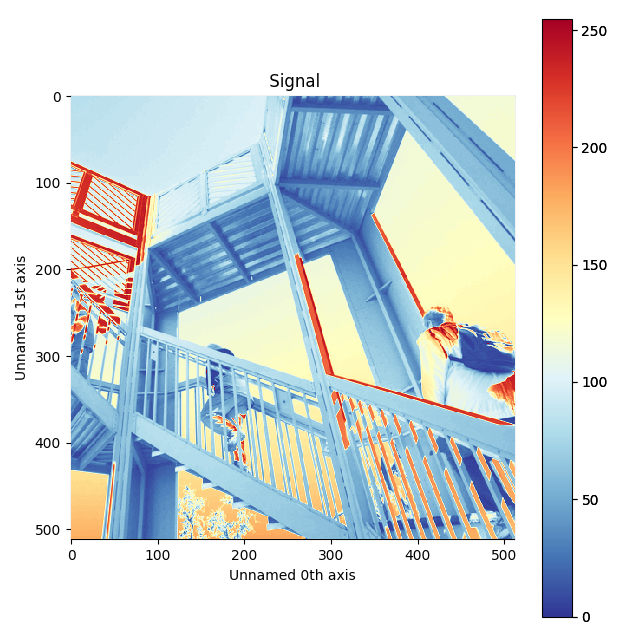Custom colormap and switched off scalebar in an image.

New in version 1.4: `norm` keyword argument

The `norm` keyword argument can be used to select between linear, logarithmic or custom (using a matplotlib norm) intensity scale. The default, “auto”, automatically selects a logarithmic scale when plotting a power spectrum.

New in version 1.6: `autoscale` keyword argument

The `autoscale` keyword argument can be used to specify which axis limits are reset when the data or navigation indices change. It can take any combinations of the following characters:

• `'x'`: to reset the horizontal axes

• `'y'`: to reset the vertical axes

• `'v'`: to reset the contrast of the image according to `vmin` and `vmax`

By default (`autoscale='v'`), only the contrast of the image will be reset automatically. For example, to reset the extent of the image (x and y) to their maxima but not the contrast, use `autoscale='xy'`; To reset all limits, including the contrast of the image, use `autoscale='xyv'`:

```>>> import numpy as np
>>> img = hs.signals.Signal2D(np.arange(10*10*10).reshape(10, 10, 10))
>>> img.plot(autoscale='xyv')
```

When plotting using divergent colormaps, if `centre_colormap` is `True` (default) the contrast is automatically adjusted so that zero corresponds to the center of the colormap (usually white). This can be useful e.g. when displaying images that contain pixels with both positive and negative values.

The following example shows the effect of centring the color map:

```>>> x = np.linspace(-2 * np.pi, 2 * np.pi, 128)
>>> xx, yy = np.meshgrid(x, x)
>>> data1 = np.sin(xx * yy)
>>> data2 = data1.copy()
>>> data2[data2 < 0] /= 4
>>> im = hs.signals.Signal2D([data1, data2])
>>> hs.plot.plot_images(im, cmap="RdBu", tight_layout=True)
```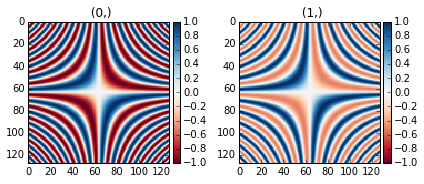Divergent color map with `Centre colormap` enabled (default).

The same example with the feature disabled:

```>>> x = np.linspace(-2 * np.pi, 2 * np.pi, 128)
>>> xx, yy = np.meshgrid(x, x)
>>> data1 = np.sin(xx * yy)
>>> data2 = data1.copy()
>>> data2[data2 < 0] /= 4
>>> im = hs.signals.Signal2D([data1, data2])
>>> hs.plot.plot_images(im, centre_colormap=False,
...                     cmap="RdBu", tight_layout=True)
```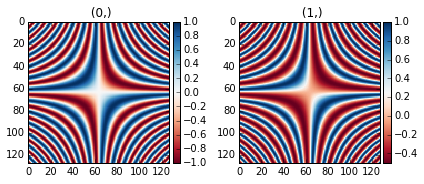Divergent color map with `centre_colormap` disabled.

## Customizing the “navigator”¶

New in version 1.1.2: Passing keyword arguments to the navigator plot.

The navigator can be customised by using the `navigator_kwds` argument. For example, in case of a image navigator, all image plot arguments mentioned in the section Customising image plot can be passed as a dictionary to the `navigator_kwds` argument:

```>>> import numpy as np
>>> import scipy
>>> im = hs.signals.Signal2D(scipy.misc.ascent())
>>> ims = hs.signals.BaseSignal(np.random.rand(15,13)).T * im
>>> ims.plot(colorbar=False,
...          scalebar=False,
...          axes_ticks=False,
...          cmap='viridis',
...          navigator_kwds=dict(colorbar=True,
...                              scalebar_color='red',
...                              cmap='Blues',
...                              axes_ticks=False)
...          )
```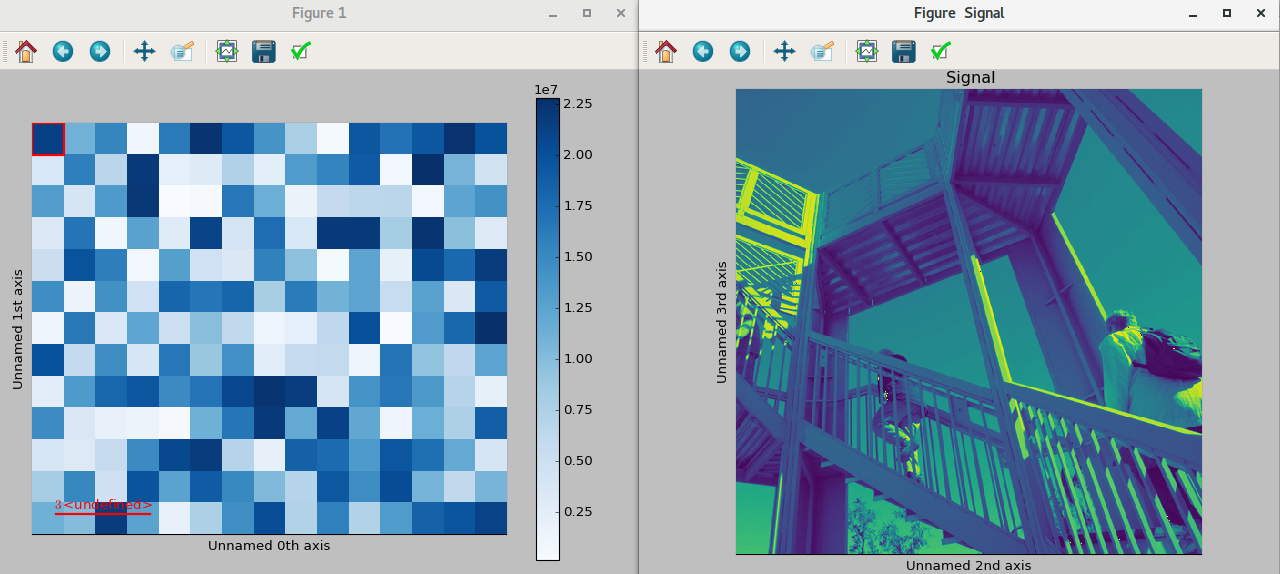Custom different options for both signal and navigator image plots

```>>> #Download the data (130MB)
>>> from urllib.request import urlretrieve, urlopen
>>> from zipfile import ZipFile
>>> files = urlretrieve("https://www.dropbox.com/s/s7cx92mfh2zvt3x/"
...                     "HyperSpy_demos_EDX_SEM_files.zip?raw=1",
...                     "./HyperSpy_demos_EDX_SEM_files.zip")
>>> with ZipFile("HyperSpy_demos_EDX_SEM_files.zip") as z:
>>>     z.extractall()
```

Note

Note

The sample and the data used in this chapter are described in P. Burdet, et al., Acta Materialia, 61, p. 3090-3098 (2013) (see abstract).

Stack of 2D images can be imported as an 3D image and plotted with a slider instead of the 2D navigator as in the previous example.

```>>> img = hs.load('Ni_superalloy_0*.tif', stack=True)
>>> img.plot(navigator='slider')
```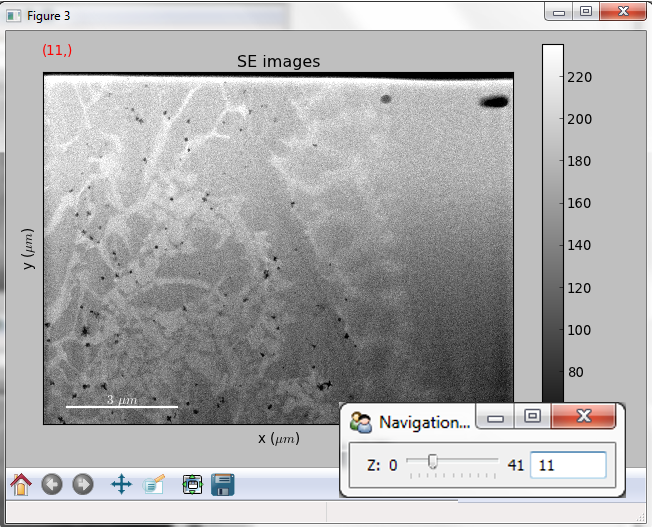Visualisation of a 3D image with a slider.

A stack of 2D spectrum images can be imported as a 3D spectrum image and plotted with sliders.

```>>> s = hs.load('Ni_superalloy_0*.rpl', stack=True).as_signal1D(0)
>>> s.plot()
```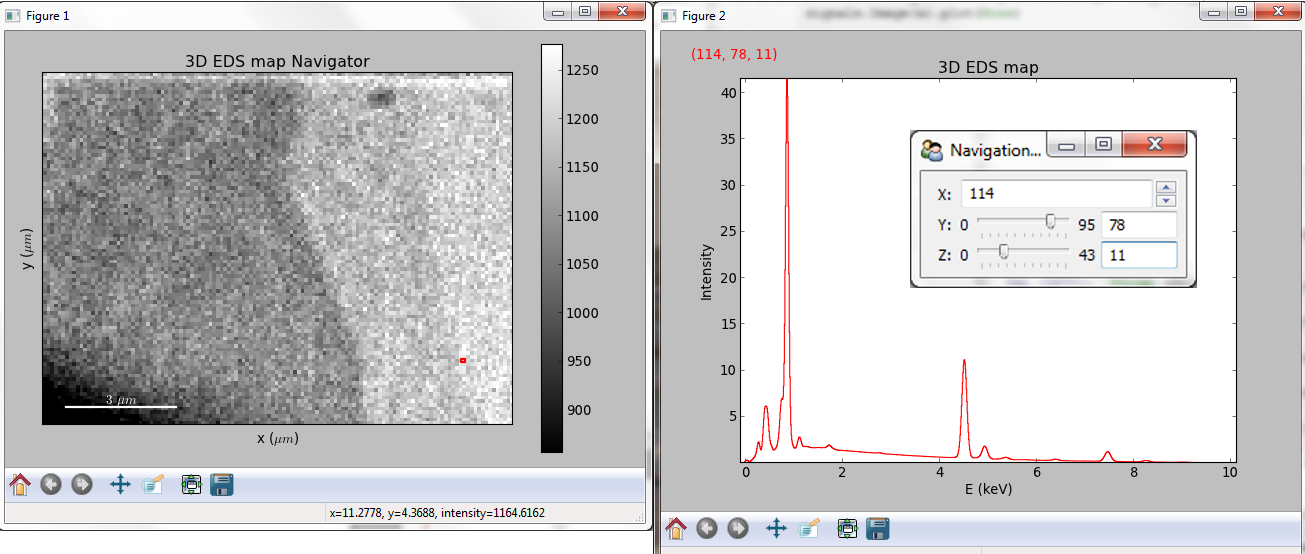Visualisation of a 3D spectrum image with sliders.

If the 3D images has the same spatial dimension as the 3D spectrum image, it can be used as an external signal for the navigator.

```>>> im = hs.load('Ni_superalloy_0*.tif', stack=True)
>>> #Rebin the image
>>> im = im.rebin([dim, dim, dim])
>>> s.plot(navigator=im)
```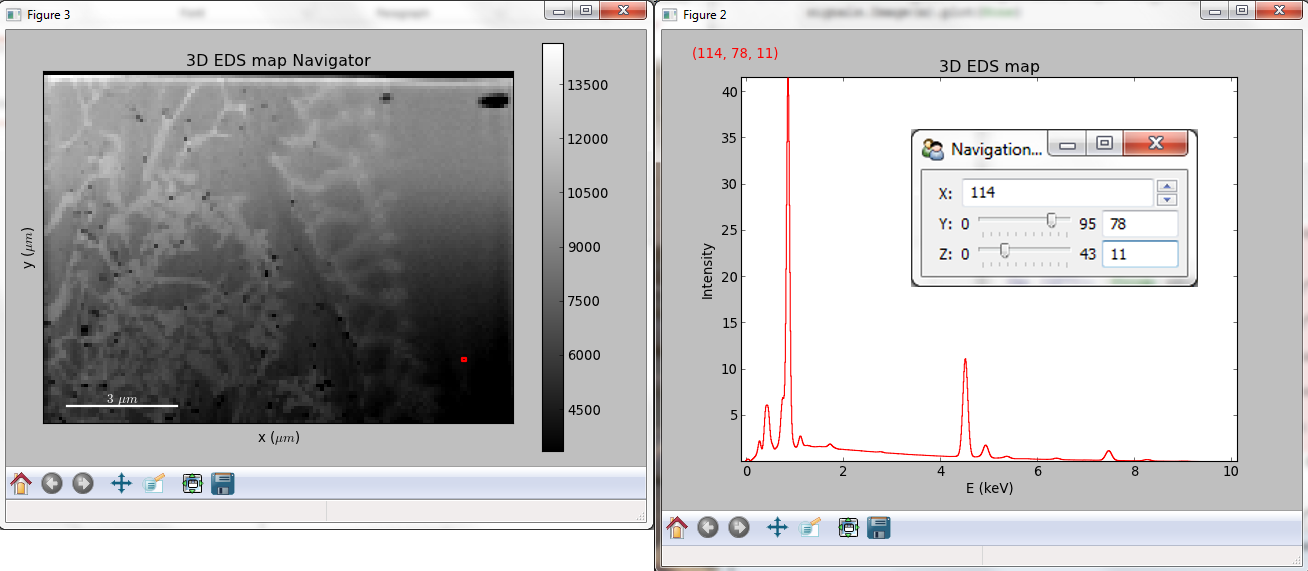Visualisation of a 3D spectrum image. The navigator is an external signal.

The 3D spectrum image can be transformed in a stack of spectral images for an alternative display.

```>>> imgSpec = hs.load('Ni_superalloy_0*.rpl', stack=True)
>>> imgSpec.plot(navigator='spectrum')
```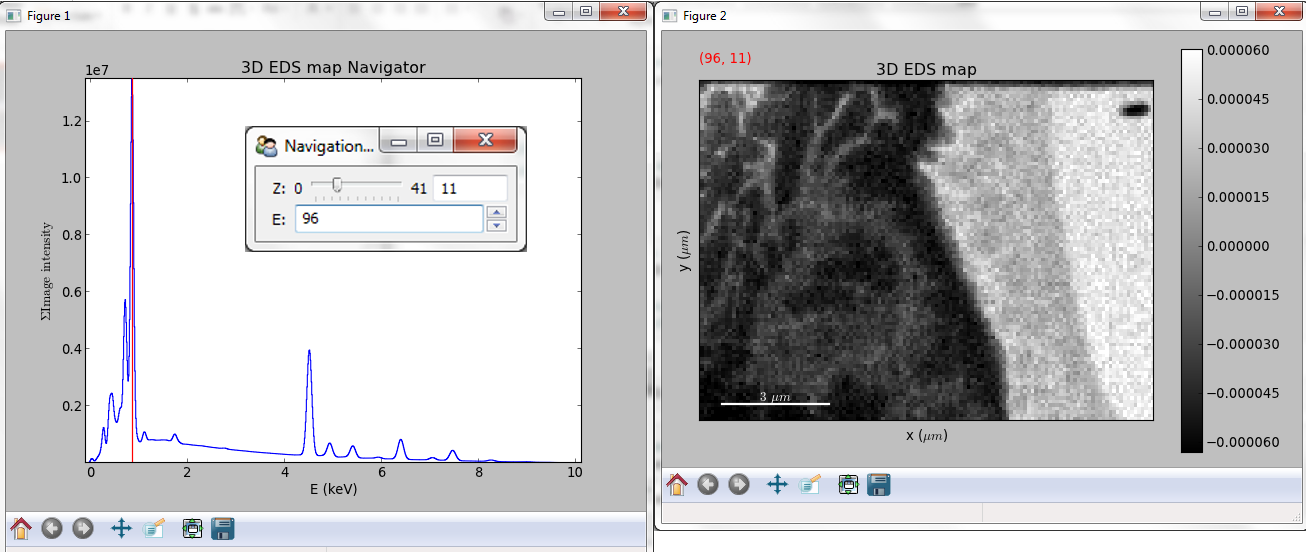Visualisation of a stack of 2D spectral images.

An external signal (e.g. a spectrum) can be used as a navigator, for example the “maximum spectrum” for which each channel is the maximum of all pixels.

```>>> imgSpec = hs.load('Ni_superalloy_0*.rpl', stack=True)
>>> specMax = imgSpec.max(-1).max(-1).max(-1).as_signal1D(0)
>>> imgSpec.plot(navigator=specMax)
```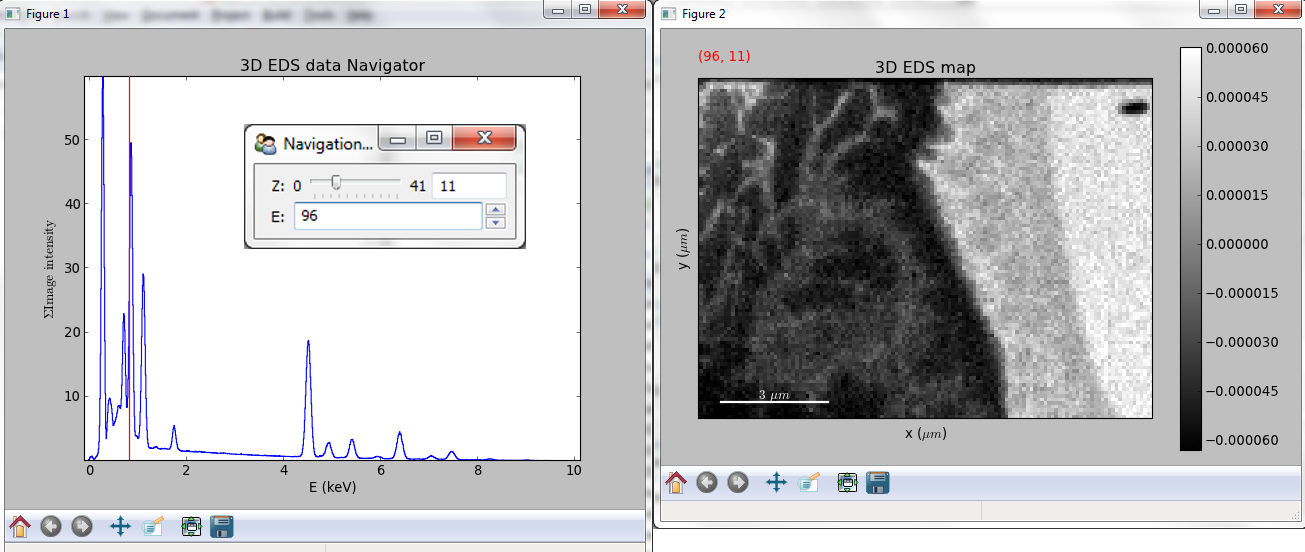Visualisation of a stack of 2D spectral images. The navigator is the “maximum spectrum”.

Lastly, if no navigator is needed, “navigator=None” can be used.

## Using Mayavi to visualize 3D data¶

```>>> from urllib.request import urlretrieve
>>> url = 'http://cook.msm.cam.ac.uk//~hyperspy//EDS_tutorial//'
>>> urlretrieve(url + 'Ni_La_intensity.hdf5', 'Ni_La_intensity.hdf5')
```

Note

Although HyperSpy does not currently support plotting when signal_dimension is greater than 2, Mayavi can be used for this purpose.

In the following example we also use scikit-image for noise reduction. More details about `get_lines_intensity()` method can be found in EDS lines intensity.

```>>> from mayavi import mlab
>>> mlab.figure()
>>> mlab.contour3d(ni.data, contours=)
>>> mlab.outline(color=(0, 0, 0))
```

Note

Note

The sample and the data used in this chapter are described in P. Burdet, et al., Ultramicroscopy, 148, p. 158-167 (2015).

## Plotting multiple signals¶

HyperSpy provides three functions to plot multiple signals (spectra, images or other signals): `plot_images()`, `plot_spectra()`, and `plot_signals()` in the `utils.plot` package.

### Plotting several images¶

`plot_images()` is used to plot several images in the same figure. It supports many configurations and has many options available to customize the resulting output. The function returns a list of matplotlib axes, which can be used to further customize the figure. Some examples are given below. Plots generated from another installation may look slightly different due to `matplotlib` GUI backends and default font sizes. To change the font size globally, use the command ```matplotlib.rcParams.update({'font .size': 8})```.

New in version 1.5: Add support for plotting `BaseSignal` with navigation dimension 2 and signal dimension 0.

A common usage for `plot_images()` is to view the different slices of a multidimensional image (a hyperimage):

```>>> import scipy
>>> image = hs.signals.Signal2D([scipy.misc.ascent()]*6)
>>> angles = hs.signals.BaseSignal(range(10,70,10))
>>> image.map(scipy.ndimage.rotate, angle=angles.T, reshape=False)
>>> hs.plot.plot_images(image, tight_layout=True)
```

This example is explained in Signal iterator.

By default, `plot_images()` will attempt to auto-label the images based on the Signal titles. The labels (and title) can be customized with the suptitle and label arguments. In this example, the axes labels and the ticks are also disabled with axes_decor:

```>>> import scipy
>>> image = hs.signals.Signal2D([scipy.misc.ascent()]*6)
>>> angles = hs.signals.BaseSignal(range(10,70,10))
>>> image.map(scipy.ndimage.rotate, angle=angles.T, reshape=False)
>>> hs.plot.plot_images(
...     image, suptitle='Turning Ascent', axes_decor='off',
...     label=['Rotation {}\$^\degree\$'.format(angles.data[i]) for
...            i in range(angles.data.shape)], colorbar=None)
```

`plot_images()` can also be used to easily plot a list of Images, comparing different Signals, including RGB images. This example also demonstrates how to wrap labels using labelwrap (for preventing overlap) and using a single colorbar for all the Images, as opposed to multiple individual ones:

```>>> import scipy
>>> import numpy as np
>>>
>>> # load red channel of raccoon as an image
>>> image0 = hs.signals.Signal2D(scipy.misc.face()[:,:,0])
>>> image0.metadata.General.title = 'Rocky Raccoon - R'
>>>
>>> # load ascent into a length 6 hyper-image
>>> image1 = hs.signals.Signal2D([scipy.misc.ascent()]*6)
>>> angles = hs.signals.BaseSignal(np.arange(10,70,10)).T
>>> image1.map(scipy.ndimage.rotate, angle=angles,
...            show_progressbar=False, reshape=False)
>>> image1.data = np.clip(image1.data, 0, 255)  # clip data to int range
>>>
>>> # load green channel of raccoon as an image
>>> image2 = hs.signals.Signal2D(scipy.misc.face()[:,:,1])
>>> image2.metadata.General.title = 'Rocky Raccoon - G'
>>>
>>> # load rgb image of the raccoon
>>> rgb = hs.signals.Signal1D(scipy.misc.face())
>>> rgb.change_dtype("rgb8")
>>> rgb.metadata.General.title = 'Raccoon - RGB'
>>>
>>> images = [image0, image1, image2, rgb]
>>> for im in images:
...     ax = im.axes_manager.signal_axes
...     ax.name, ax.name = 'x', 'y'
...     ax.units, ax.units = 'mm', 'mm'
>>> hs.plot.plot_images(images, tight_layout=True,
...                     colorbar='single', labelwrap=20)
```

Data files used in the following example can be downloaded using (These data are described in [Rossouw2015].

```>>> #Download the data (1MB)
>>> from urllib.request import urlretrieve, urlopen
>>> from zipfile import ZipFile
>>> files = urlretrieve("https://www.dropbox.com/s/ecdlgwxjq04m5mx/"
...                     "HyperSpy_demos_EDS_TEM_files.zip?raw=1",
...                     "./HyperSpy_demos_EDX_TEM_files.zip")
>>> with ZipFile("HyperSpy_demos_EDX_TEM_files.zip") as z:
>>>     z.extractall()
```

Another example for this function is plotting EDS line intensities see EDS chapter. One can use the following commands to get a representative figure of the X-ray line intensities of an EDS spectrum image. This example also demonstrates changing the colormap (with cmap), adding scalebars to the plots (with scalebar), and changing the padding between the images. The padding is specified as a dictionary, which is used to call subplots_adjust method of matplotlib (see documentation).

```>>> si_EDS = hs.load("core_shell.hdf5")
>>> im = si_EDS.get_lines_intensity()
>>> hs.plot.plot_images(im,
...     tight_layout=True, cmap='RdYlBu_r', axes_decor='off',
...     colorbar='single', vmin='1th', vmax='99th', scalebar='all',
...     scalebar_color='black', suptitle_fontsize=16,
...              'right':0.85, 'wspace':0.20, 'hspace':0.10})
```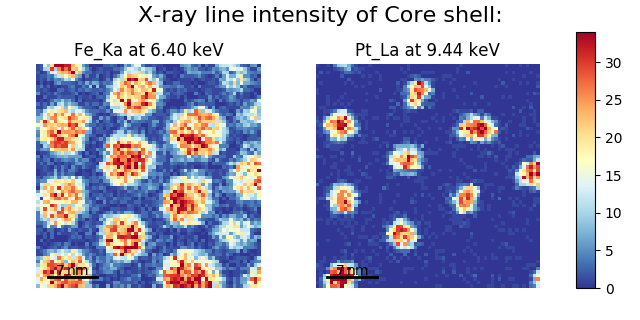Using `plot_images()` to plot the output of `get_lines_intensity()`.

Note

This padding can also be changed interactively by clicking on thebutton in the GUI (button may be different when using different graphical backends).

Finally, the `cmap` option of `plot_images()` supports iterable types, allowing the user to specify different colormaps for the different images that are plotted by providing a list or other generator:

```>>> si_EDS = hs.load("core_shell.hdf5")
>>> im = si_EDS.get_lines_intensity()
>>> hs.plot.plot_images(im,
>>>    tight_layout=True, cmap=['viridis', 'plasma'], axes_decor='off',
>>>    colorbar='multi', vmin='1th', vmax='99th', scalebar=,
>>>    scalebar_color='white', suptitle_fontsize=16)
```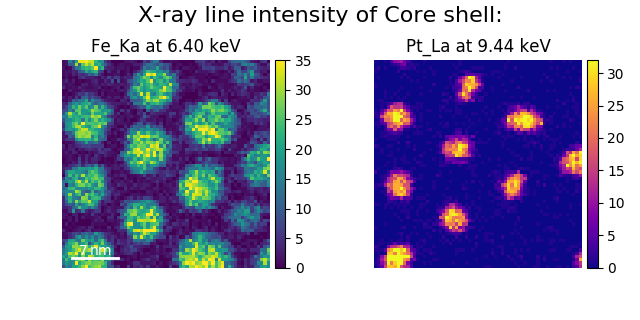Using `plot_images()` to plot the output of `get_lines_intensity()` using a unique colormap for each image.

The `cmap` argument can also be given as `'mpl_colors'`, and as a result, the images will be plotted with colormaps generated from the default `matplotlib` colors, which is very helpful when plotting multiple spectral signals and their relative intensities (such as the results of a `decomposition()` analysis). This example uses `plot_spectra()`, which is explained in the next section.

```>>> si_EDS = hs.load("core_shell.hdf5")
>>> si_EDS.change_dtype('float')
>>> si_EDS.decomposition(True, algorithm='NMF', output_dimension=3)
>>> factors = si_EDS.get_decomposition_factors()
>>>
>>> # the first factor is a very strong carbon background component, so we
>>> # normalize factor intensities for easier qualitative comparison
>>> for f in factors:
>>>     f.data /= f.data.max()
>>>
>>>
>>>
>>> # add some lines to nicely label the peak positions
>>> plt.axvline(6.403, c='C2', ls=':', lw=0.5)
>>> plt.text(x=6.503, y=0.85, s='Fe-K\$_\\alpha\$', color='C2')
>>> plt.axvline(9.441, c='C1', ls=':', lw=0.5)
>>> plt.text(x=9.541, y=0.85, s='Pt-L\$_\\alpha\$', color='C1')
>>> plt.axvline(2.046, c='C1', ls=':', lw=0.5)
>>> plt.text(x=2.146, y=0.85, s='Pt-M', color='C1')
>>> plt.axvline(8.040, ymax=0.8, c='k', ls=':', lw=0.5)
>>> plt.text(x=8.14, y=0.35, s='Cu-K\$_\\alpha\$', color='k')
>>>
>>>             axes_decor='off', per_row=1,
>>>             label=['Background', 'Pt core', 'Fe shell'],
>>>             scalebar=, scalebar_color='white',
>>>                      'left': 0.05, 'right':0.78})
```Using `plot_images()` with `cmap='mpl_colors'` together with `plot_spectra()` to visualize the output of a non-negative matrix factorization of the EDS data.

Note

Because it does not make sense, it is not allowed to use a list or other iterable type for the `cmap` argument together with `'single'` for the `colorbar` argument. Such an input will cause a warning and instead set the `colorbar` argument to `None`.

### Plotting several spectra¶

`plot_spectra()` is used to plot several spectra in the same figure. It supports different styles, the default being “overlap”.

New in version 1.5: Add support for plotting `BaseSignal` with navigation dimension 1 and signal dimension 0.

In the following example we create a list of 9 single spectra (gaussian functions with different sigma values) and plot them in the same figure using `plot_spectra()`. Note that, in this case, the legend labels are taken from the individual spectrum titles. By clicking on the legended line, a spectrum can be toggled on and off.

```>>> s = hs.signals.Signal1D(np.zeros((200)))
>>> s.axes_manager.offset = -10
>>> s.axes_manager.scale = 0.1
>>> m = s.create_model()
>>> g = hs.model.components1D.Gaussian()
>>> m.append(g)
>>> gaussians = []
>>> labels = []

>>> for sigma in range(1, 10):
...         g.sigma.value = sigma
...         gs = m.as_signal()
...         gs.metadata.General.title = "sigma=%i" % sigma
...         gaussians.append(gs)
...
>>> hs.plot.plot_spectra(gaussians,legend='auto')
<matplotlib.axes.AxesSubplot object at 0x4c28c90>
```

Another style, “cascade”, can be useful when “overlap” results in a plot that is too cluttered e.g. to visualize changes in EELS fine structure over a line scan. The following example shows how to plot a cascade style figure from a spectrum, and save it in a file:

```>>> import scipy.misc
>>> s = hs.signals.Signal1D(scipy.misc.ascent()[100:160:10])
```

The “cascade” style has a padding option. The default value, 1, keeps the individual plots from overlapping. However in most cases a lower padding value can be used, to get tighter plots.

Using the color argument one can assign a color to all the spectra, or specific colors for each spectrum. In the same way, one can also assign the line style and provide the legend labels:

```>>> import scipy.misc
>>> s = hs.signals.Signal1D(scipy.misc.ascent()[100:160:10])
>>> color_list = ['red', 'red', 'blue', 'blue', 'red', 'red']
>>> line_style_list = ['-','--','steps','-.',':','-']
>>> line_style=line_style_list,legend='auto')
```

A simple extension of this functionality is to customize the colormap that is used to generate the list of colors. Using a list comprehension, one can generate a list of colors that follows a certain colormap:

```>>> import scipy.misc
>>> fig, axarr = plt.subplots(1,2)
>>> s1 = hs.signals.Signal1D(scipy.misc.ascent()[100:160:10])
>>> s2 = hs.signals.Signal1D(scipy.misc.ascent()[200:260:10])
>>> hs.plot.plot_spectra(s1,
...                         color=[plt.cm.RdBu(i/float(len(s1)-1))
...                                for i in range(len(s1))],
...                         ax=axarr,
...                         fig=fig)
>>> hs.plot.plot_spectra(s2,
...                         color=[plt.cm.summer(i/float(len(s1)-1))
...                                for i in range(len(s1))],
...                         ax=axarr,
...                         fig=fig)
>>> axarr.set_xlabel('RdBu (colormap)')
>>> axarr.set_xlabel('summer (colormap)')
>>> fig.canvas.draw()
```

There are also two other styles, “heatmap” and “mosaic”:

```>>> import scipy.misc
>>> s = hs.signals.Signal1D(scipy.misc.ascent()[100:160:10])
>>> hs.plot.plot_spectra(s, style='heatmap')
```
```>>> import scipy.misc
>>> s = hs.signals.Signal1D(scipy.misc.ascent()[100:120:10])
>>> hs.plot.plot_spectra(s, style='mosaic')
```

For the “heatmap” style, different matplotlib color schemes can be used:

```>>> import matplotlib.cm
>>> import scipy.misc
>>> s = hs.signals.Signal1D(scipy.misc.ascent()[100:120:10])
>>> ax = hs.plot.plot_spectra(s, style="heatmap")
>>> ax.images.set_cmap(matplotlib.cm.plasma)
```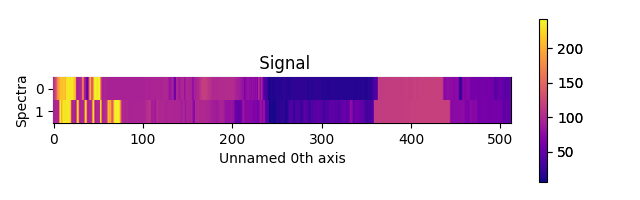Figure generated by `plot_spectra()` using the heatmap style showing how to customise the color map.

Any parameter that can be passed to matplotlib.pyplot.figure can also be used with plot_spectra() to allow further customization (when using the “overlap”, “cascade”, or “mosaic” styles). In the following example, dpi, facecolor, frameon, and num are all parameters that are passed directly to matplotlib.pyplot.figure as keyword arguments:

```>>> import scipy.misc
>>> s = hs.signals.Signal1D(scipy.misc.ascent()[100:160:10])
>>> legendtext = ['Plot 0', 'Plot 1', 'Plot 2', 'Plot 3',
...               'Plot 4', 'Plot 5']
...     facecolor='lightblue', frameon=True, num=5)
>>> plt.draw()
```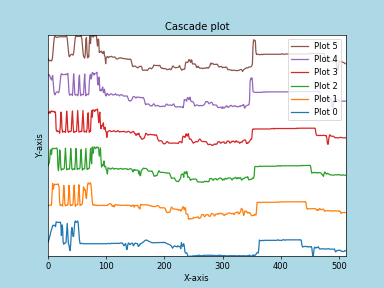Customising the figure with keyword arguments.

The function returns a matplotlib ax object, which can be used to customize the figure:

```>>> import scipy.misc
>>> s = hs.signals.Signal1D(scipy.misc.ascent()[100:160:10])
>>> plt.draw()
```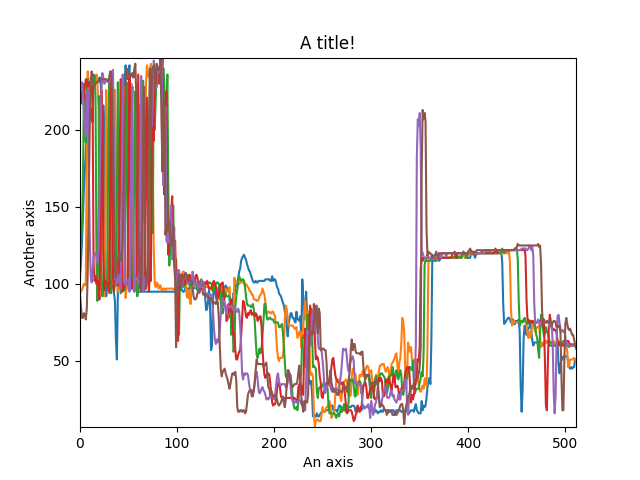Customising the figure by setting the matplotlib axes properties.

A matplotlib ax and fig object can also be specified, which can be used to put several subplots in the same figure. This will only work for “cascade” and “overlap” styles:

```>>> import scipy.misc
>>> fig, axarr = plt.subplots(1,2)
>>> s1 = hs.signals.Signal1D(scipy.misc.ascent()[100:160:10])
>>> s2 = hs.signals.Signal1D(scipy.misc.ascent()[200:260:10])
...                      color='blue', ax=axarr, fig=fig)
...                      color='red', ax=axarr, fig=fig)
>>> fig.canvas.draw()
```

### Plotting profiles interactively¶

Spectra or line profile can be plotted interactively on the same figure using the `plot_spectra()` function. For example, profiles obtained from different Signal2D using the `Line2DROI` ROI can be plotted interactively:

```>>> im0 = hs.datasets.example_signals.reference_hologram()
>>> im1 = hs.datasets.example_signals.object_hologram()
>>> im0.plot()
>>> im1.plot()
>>> # Create the ROI
>>> line_profile = hs.roi.Line2DROI(400, 250, 220, 600)
>>> # Obtain the signals to plot by "slicing" the signals with the ROI
>>> line0 = line_profile.interactive(im0)
>>> line1 = line_profile.interactive(im1)
>>> # Plotting the profile on the same figure
>>> hs.plot.plot_spectra([line0, line1])
```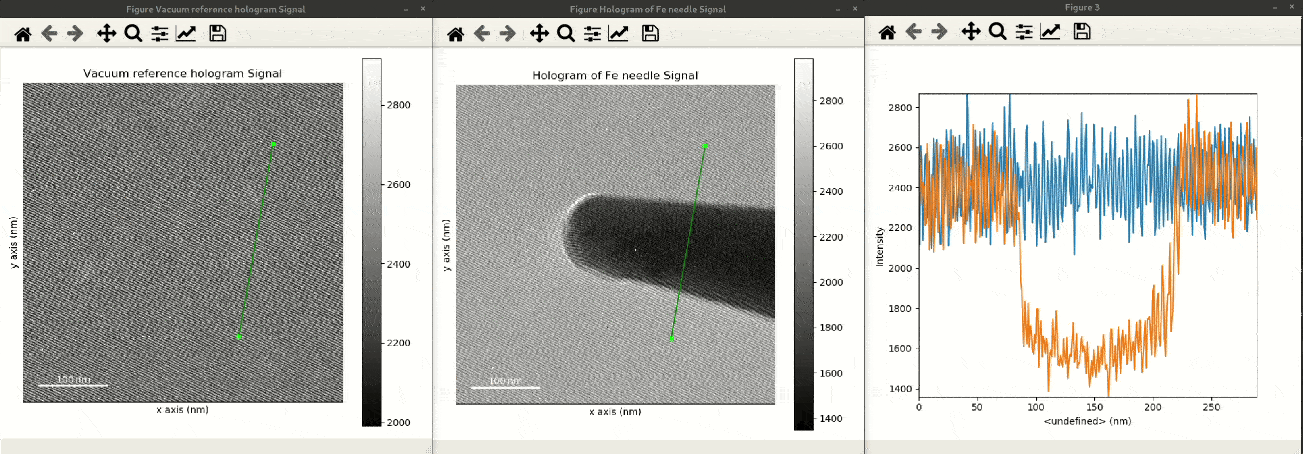Plotting profiles from different images interactively.

#### Plotting several signals¶

`plot_signals()` is used to plot several signals at the same time. By default the navigation position of the signals will be synced, and the signals must have the same dimensions. To plot two spectra at the same time:

```>>> import scipy.misc
>>> s1 = hs.signals.Signal1D(scipy.misc.face()).as_signal1D(0).inav[:,:3]
>>> s2 = s1.deepcopy()*-1
>>> hs.plot.plot_signals([s1, s2])
```The `plot_signals()` plots several signals with optional synchronized navigation.

The navigator can be specified by using the navigator argument, where the different options are “auto”, None, “spectrum”, “slider” or Signal. For more details about the different navigators, see the navigator options. To specify the navigator:

```>>> import scipy.misc
>>> s1 = hs.signals.Signal1D(scipy.misc.face()).as_signal1D(0).inav[:,:3]
>>> s2 = s1.deepcopy()*-1
>>> hs.plot.plot_signals([s1, s2], navigator="slider")
```

Navigators can also be set differently for different plots using the navigator_list argument. Where the navigator_list be the same length as the number of signals plotted, and only contain valid navigator options. For example:

```>>> import scipy.misc
>>> s1 = hs.signals.Signal1D(scipy.misc.face()).as_signal1D(0).inav[:,:3]
>>> s2 = s1.deepcopy()*-1
>>> s3 = hs.signals.Signal1D(np.linspace(0,9,9).reshape([3,3]))
>>> hs.plot.plot_signals([s1, s2], navigator_list=["slider", s3])
```

Several signals can also be plotted without syncing the navigation by using sync=False. The navigator_list can still be used to specify a navigator for each plot:

```>>> import scipy.misc
>>> s1 = hs.signals.Signal1D(scipy.misc.face()).as_signal1D(0)[:,:3]
>>> s2 = s1.deepcopy()*-1
>>> hs.plot.plot_signals([s1, s2], sync=False,
...                      navigator_list=["slider", "slider"])
```

## Markers¶

HyperSpy provides an easy access to the main marker of matplotlib. The markers can be used in a static way

```>>> import scipy.misc
>>> im = hs.signals.Signal2D(scipy.misc.ascent())
>>> m = hs.plot.markers.rectangle(x1=150, y1=100,
...                               x2=400, y2=400, color='red')
```

By providing an array of positions, the marker can also change position when navigating the signal. In the following example, the local maxima are displayed for each R, G and B channel of a colour image.

```>>> from skimage.feature import peak_local_max
>>> import scipy.misc
>>> ims = hs.signals.BaseSignal(scipy.misc.face()).as_signal2D([1,2])
>>> index = np.array([peak_local_max(im.data, min_distance=100,
...                                  num_peaks=4)
...                   for im in ims])
>>> for i in range(4):
...     m = hs.plot.markers.point(x=index[:, i, 1],
...                               y=index[:, i, 0], color='red')
```

The markers can be added to the navigator as well. In the following example, each slice of a 2D spectrum is tagged with a text marker on the signal plot. Each slice is indicated with the same text on the navigator.

```>>> s = hs.signals.Signal1D(np.arange(100).reshape([10,10]))
>>> s.plot(navigator='spectrum')
>>> for i in range(s.axes_manager.shape):
...     m = hs.plot.markers.text(y=s.sum(-1).data[i]+5,
...                              x=i, text='abcdefghij'[i])
>>> x = s.axes_manager.shape[-1]/2 #middle of signal plot
>>> m = hs.plot.markers.text(x=x, y=s.isig[x].data+2,
...                          text=[i for i in 'abcdefghij'])
```

New in version 1.2: Permanent markers.

These markers can also be permanently added to a signal, which is saved in `metadata.Markers`:

```>>> s = hs.signals.Signal2D(np.arange(100).reshape(10, 10))
>>> marker = hs.markers.point(5, 9)
└── point = <marker.Point, point (x=5,y=9,color=black,size=20)>
>>> s.plot()
```

Markers can be removed by deleting them from the metadata

```>>> s = hs.signals.Signal2D(np.arange(100).reshape(10, 10))
>>> marker = hs.markers.point(5, 9)
└── point = <marker.Point, point (x=5,y=9,color=black,size=20)>
```

To suppress plotting of permanent markers, use plot_markers=False when calling s.plot:

```>>> s = hs.signals.Signal2D(np.arange(100).reshape(10, 10))
>>> marker = hs.markers.point(5, 9)
>>> s.plot(plot_markers=False)
```

If the signal has a navigation dimension, the markers can be made to change as a function of the navigation index. For a signal with 1 navigation axis:

```>>> s = hs.signals.Signal2D(np.arange(300).reshape(3, 10, 10))
>>> marker = hs.markers.point((5, 1, 2), (9, 8, 1), color='red')
```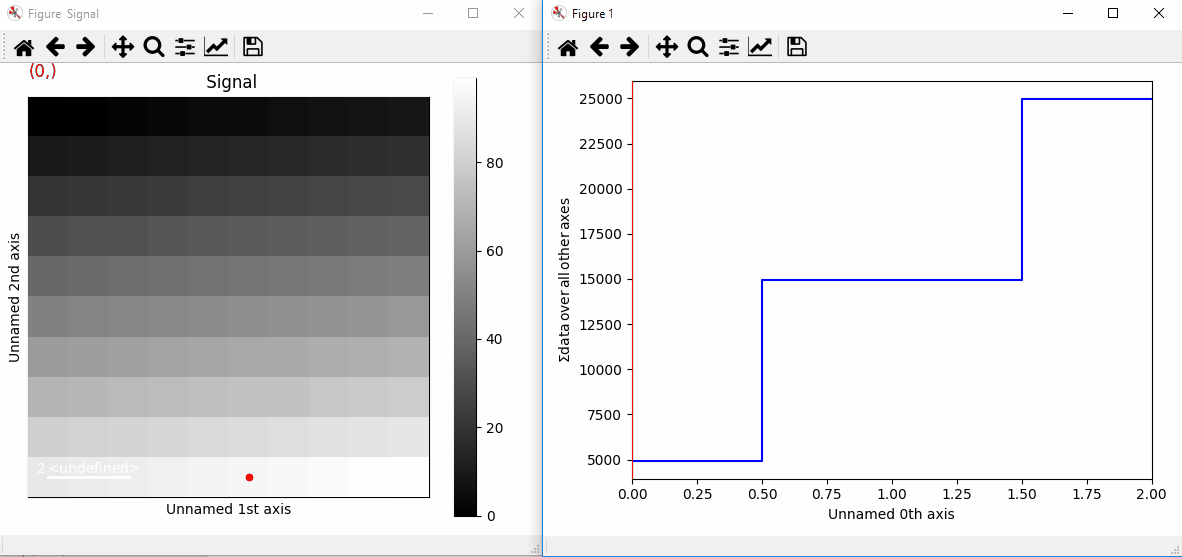Plotting with markers that change with the navigation index.

Or for a signal with 2 navigation axes:

```>>> s = hs.signals.Signal2D(np.arange(400).reshape(2, 2, 10, 10))
>>> marker = hs.markers.point(((5, 1), (1, 2)), ((2, 6), (9, 8)))
```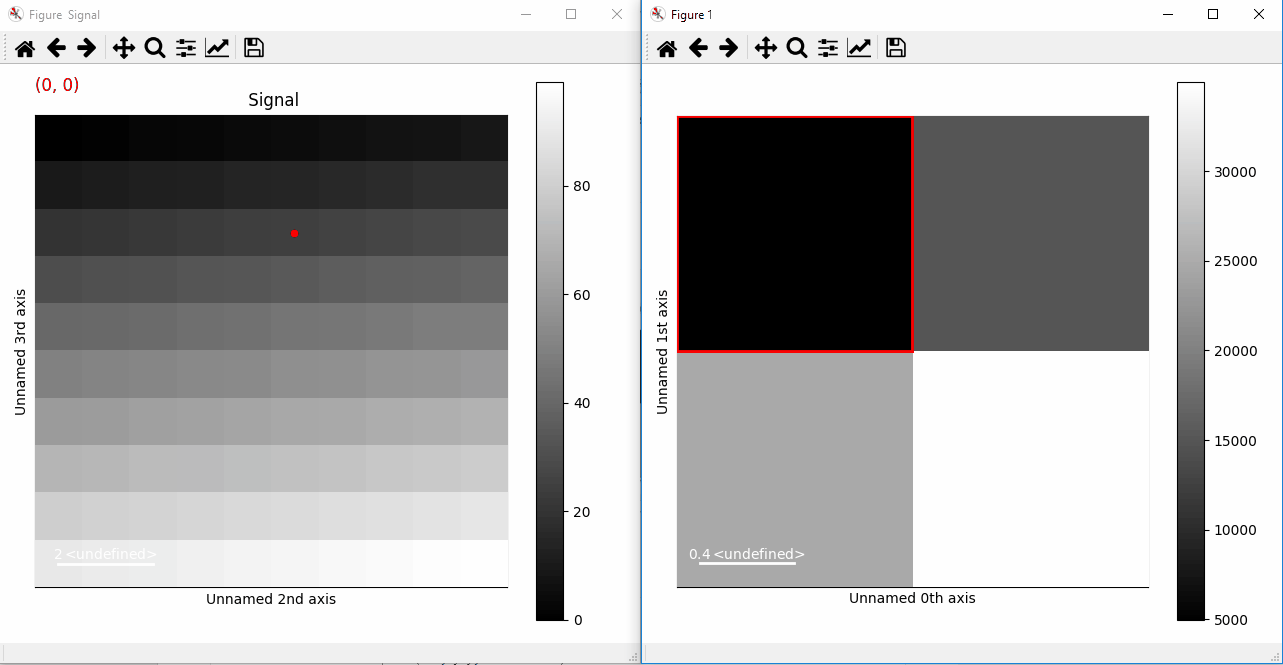Plotting with markers that change with the two-dimensional navigation index.

This can be extended to 4 (or more) navigation dimensions:

```>>> s = hs.signals.Signal2D(np.arange(1600).reshape(2, 2, 2, 2, 10, 10))
>>> x = np.arange(16).reshape(2, 2, 2, 2)
>>> y = np.arange(16).reshape(2, 2, 2, 2)
>>> marker = hs.markers.point(x=x, y=y, color='red')
```

New in version 1.2: `markers` keyword arguments can take an iterable in addition to single marker.

If you want to add a large amount of markers at the same time we advise to add them as an iterable (list, tuple, …), which will be much faster:

```>>> from numpy.random import random
>>> s = hs.signals.Signal2D(np.arange(300).reshape(3, 10, 10))
>>> markers = (hs.markers.point(tuple(random()*10 for i in range(3)),
...                             tuple(random()*10 for i in range(3)),
...                             size=30, color=np.random.rand(3,1))
...            for i in range(500))
```Plotting many markers with an iterable so they change with the navigation index.

This can also be done using different types of markers

```>>> from numpy.random import random
>>> s = hs.signals.Signal2D(np.arange(300).reshape(3, 10, 10))
>>> markers = []
>>> for i in range(200):
...     markers.append(hs.markers.horizontal_line(
...         tuple(random()*10 for i in range(3)),
...         color=np.random.rand(3,1)))
...     markers.append(hs.markers.vertical_line(
...         tuple(random()*10 for i in range(3)),
...         color=np.random.rand(3,1)))
...     markers.append(hs.markers.point(
...         tuple(random()*10 for i in range(3)),
...         tuple(random()*10 for i in range(3)),
...         color=np.random.rand(3,1)))
...     markers.append(hs.markers.text(
...         x=tuple(random()*10 for i in range(3)),
...         y=tuple(random()*10 for i in range(3)),
...         text=tuple("sometext" for i in range(3))))
```Plotting many types of markers with an iterable so they change with the navigation index.

Permanent markers are stored in the HDF5 file if the signal is saved:

```>>> s = hs.signals.Signal2D(np.arange(100).reshape(10, 10))
>>> marker = hs.markers.point(2, 1, color='red')